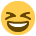# Can get my head around this financial performance question..PLZ help

I have given:
budgeted: production 20000 units, DM: 40000kg - 300000, DL 10000hrs - 60000, Total costs 360000
actual: production 23000 units, DM 48000kg - 336000, DL 14000hrs - 81200, Total costs 417200

Have to calculate the relevant direct cost variances and use them to reconcile the standard costs, but its asks to calculate the standard costs and quantities for one unit.

Also, there is a idle time for labour for 200hrs in August.

Plz someone help me...I cant get my head around why my variances doesnt match if I prepare the standard costs as for one unit and when I use for whole production.
:confused1:

• Hi there,

I will give you a starting hand, your standard cost card for 1 unit would look like: -

Materials 2kgs @ £ 7.50 per Kg= 15.00
Labour 0.5 Hours @ £ 6.00 per Hour = 3.00
Total Standard Cost for 1 Unit = 18.00

You probably already have this, I will have a look a how it reconciles to actual (If I can work it out :P)
• OK, I have got this far...

Standard cost of actual production = 23,000 * £18 = £ 414,000
Variances:
Material Price 24,000 (F)
Material Usage 15,000 (A)
Labour Rate 2,800 (F)
Labour Usage 15,000 (A)
Actual Cost of Actual Production = £ 417,200

Basically, you would take the standard cost of actual production, deduct the favorable variances and add the adverse variances to reconcile back to Actual cost of Actual production. Im not sure how the idle time fits in, I think this should be an adverse variance so maybe my labour usage is incorrect. Let me know if you need explain how any of the variances were calclated.
• Further to above I have the Idle Time Variance of 1,200 (A) (200hours @ £6), Labour Usage Variance of 13,800 (A)

Labour Usage Variance calculated as Std of 11,700 hours @ £ 6 less Act of 14,000 hours @ £ 6.

Well that was fun..., and hopefully correct• I get confused with labour efficiency variance which in my case is 42000A then 200x£6.00=1200A (Idle time)

Can you plz let me know how you get 11700hrs?

I done like this : 14000 x 0.5=7000hrs x 6.00 = 42000
14000hrs x 6.00= 84000
14000hrs x 5.80 = 81200, which gives 42000A efficiency and 2800F rate
then 200hrs x 6.00 = 1200A => 42000A - 1200A=40800A but I know its wrong as it dosnt reconcile how it should be lol
• crispy wrote: »
OK, I have got this far...

Standard cost of actual production = 23,000 * £18 = £ 414,000
Variances:
Material Price 24,000 (F)
Material Usage 15,000 (A)
Labour Rate 2,800 (F)
Labour Usage 15,000 (A)
Actual Cost of Actual Production = £ 417,200

Basically, you would take the standard cost of actual production, deduct the favorable variances and add the adverse variances to reconcile back to Actual cost of Actual production. Im not sure how the idle time fits in, I think this should be an adverse variance so maybe my labour usage is incorrect. Let me know if you need explain how any of the variances were calclated.

I have exactly same results as yours excluding labour efficiency variance ;-(
• I did.....

Standard Labour Hours for Actual Production =

23,000 Units @ 0.5 Hours per Unit = 11,500 Hours
11,500 Hours + Idle Time 200 = 11,700 Hours
• crispy wrote: »
I did.....

Standard Labour Hours for Actual Production =

23,000 Units @ 0.5 Hours per Unit = 11,500 Hours
11,500 Hours + Idle Time 200 = 11,700 Hours

OMG how stupid am I :ohmy: I used hrs instead of units thats why I got all the efficiency part wrong. Been staring at numbers for a while and just couldnt get what I have done wrong lol THANK YOU SO MUCH!!!!
I think I might have to book an appointment at Specsavers• No problemo... HTH